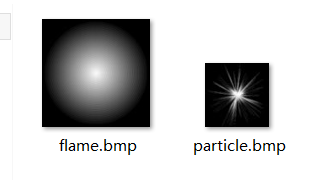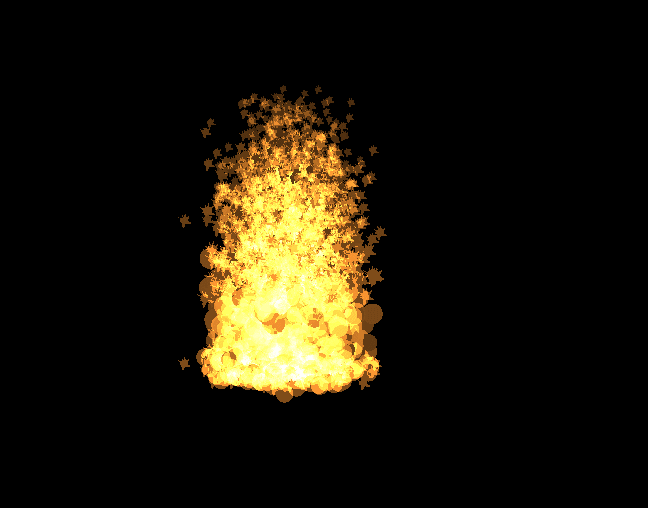# 火焰粒子系统

• 前言
• 火焰的物理模型
• 火焰粒子系统构建
• 实现效果演示

## 火焰的物理模型

1.火焰燃烧时，通常是焰心部分火焰非常旺盛，向外逐渐减少。这个特性用粒子来描述就是：通常粒子初始化区域的中心附近粒子较为密集，向外逐渐变得稀疏，呈正太分布，通过下式可以对粒子的初始位置进行赋值，使之符合正太分布的特征：

Pos(x)=12PIrexp((XiX0)22r2)
Pos(y)=0
Pos(z)=12PIrexp((ZiZ0)22r2)

Pos(y)=0

2.火焰粒子速度的初始化，粒子的速度是个非常重要的因素，需要保证它的局部随机性，又要在整体上维持火焰的外观。这里采用平均数法:

InitVeloc=(MaxVelocMinVeloc)rand_Num+MinVeloc

InitVeloc为初始速度，MaxVeloc为最大的速度，MinVeloc是最小的速度，rand_Num为[0,1]的随机数。这个公式不难理解。

3.火焰粒子的寿命初始化，中心的火焰粒子寿命长，边缘的粒子寿命短。所以初始化时火焰粒子的寿命是当前粒子到火焰中心的距离有关的，你可以是写个线性函数使之火焰的寿命随着距离的增加而减少。这里我将其简化了，初始化时设定一个中心区域的区里r，粒子到中心的距离超过这个r则寿命不变，没有超过则增加它的寿命。请注意不要将寿命和年龄混淆了！如下所示：

           dist = sqrt(Position1.x*Position1.x + Position1.z*Position1.z);
if(dist <= r)Life*= 1.3;

3.火焰粒子运动模型，对于火焰粒子来说，重力加速度几乎可以忽略，你见过那种火焰受到重力因素而掉下来的→_→。相反，空气浮力的因素对火焰的影响更大。所以我们就假设在y方向，火焰受到一个y轴正向的作用力，所以最基础的加速度向量为vec3(0.0,1.0,0.0)，浮力多少可以根据效果调整。那么收到风力的影响就直接改变这个加速度向量的x或z分量即可。

4.火焰运动过程中的大小的变化，其实我们细心的观察一下，火焰在燃烧的过程中大小的变化也是呈现一种正太分布的！一开始火焰粒子大小比较小，然后逐渐增大，当到达一定的时间后，它又逐渐变小然后消失。我们以年龄中期为分界线，那么以粒子年龄为自变量，火焰生命周期中大小的变化符合正太分布：

factor=1.0(AgeLife/2)2+1.0

Size=factorMax_size

alpha=factor

## 火焰粒子系统构建

    struct FlameParticle
{
float type;//粒子类型，分发射器和第二级粒子
glm::vec3 position;
glm::vec3 velocity;
float alpha;//alpha通道
float size;//粒子点精灵大小
float life;//寿命
};

    void Flame::GenInitLocation(FlameParticle particles[], int nums)
{
srand(time(NULL));
int n = 10;
for (int x = 0; x < nums; x++) {
glm::vec3 record(0.0f);
for (int y = 0; y < n; y++) {//生成高斯分布的粒子，中心多，外边少
record.x += (2.0f*float(rand()) / float(RAND_MAX) - 1.0f);
record.z += (2.0f*float(rand()) / float(RAND_MAX) - 1.0f);
}
record.y = center.y;
particles[x].type = PARTICLE_TYPE_LAUNCHER;
particles[x].position = record;
particles[x].velocity = DEL_VELOC*(float(rand()) / float(RAND_MAX)) + MIN_VELOC;//在最大最小速度之间随机选择
particles[x].alpha = 1.0f;
particles[x].size = INIT_SIZE;//发射器粒子大小
//在最短最长寿命之间随机选择
particles[x].lifetimeMills = (MAX_LIFE - MIN_LIFE)*(float(rand()) / float(RAND_MAX)) + MIN_LIFE;
float dist = sqrt(record.x*record.x + record.z*record.z);
}
}

#version 330 core
layout (points) in;
layout (points,max_vertices = 10) out;

in float Type0[];
in vec3 Position0[];
in vec3 Velocity0[];
in float Age0[];
in float Alpha0[];
in float Size0[];
in float Life0[];

out float Type1;
out vec3 Position1;
out vec3 Velocity1;
out float Age1;
out float Alpha1;
out float Size1;
out float Life1;

uniform float gDeltaTimeMillis;//每帧时间变化量
uniform float gTime;//总的时间变化量
uniform sampler1D gRandomTexture;
uniform float MAX_LIFE;
uniform float MIN_LIFE;
uniform vec3 MAX_VELOC;
uniform vec3 MIN_VELOC;
uniform float r;

#define PARTICLE_TYPE_LAUNCHER 0.0f
#define PARTICLE_TYPE_SHELL 1.0f

vec3 GetRandomDir(float TexCoord)
{
vec3 Dir = texture(gRandomTexture,TexCoord).xyz;
Dir -= vec3(0.5,0.5,0.5);
return Dir;
}

vec3 Rand(float TexCoord){//随机0-1
vec3 ret = texture(gRandomTexture,TexCoord).xyz;
return ret;
}

void main()
{
float Age = Age0 - gDeltaTimeMillis;
if(Type0 == PARTICLE_TYPE_LAUNCHER){//火焰发射粒子
if(Age <= 0 ){
//发射第二级粒子
Type1 = PARTICLE_TYPE_SHELL;
Position1 = Position0;
//与初始发射器一样，在最大和最小速度之间随机
Velocity1 = (MAX_VELOC-MIN_VELOC)*Rand(Age0).x+MIN_VELOC;
//寿命同上
Age1 = (MAX_LIFE-MIN_LIFE)*Rand(Age0).y + MIN_LIFE;
//求当前粒子到圆心的距离，默认中心在原点
float dist = sqrt(Position1.x*Position1.x + Position1.z*Position1.z);
//火焰的寿命在中心长一点，边缘短，这里简单以到中心的距离为标准
//r为火焰中心半径
if(dist <= r)Age1 *= 1.3;
Life1 = Age1;
Alpha1 = Alpha0;
Size1 = Size0;
EmitVertex();
EndPrimitive();
Age = (MAX_LIFE-MIN_LIFE)*Rand(Age0).z + MIN_LIFE;
}
Type1 = PARTICLE_TYPE_LAUNCHER;
Position1 = Position0;
Velocity1 = Velocity0;
Age1 = Age;
Alpha1 = Alpha0;
Size1 = Size0;
Life1 = Life0;
EmitVertex();
EndPrimitive();
}
else{//第二级粒子
if(Age >= 0){
//将时间转为以秒为单位
float DeltaTimeSecs = gDeltaTimeMillis/1000.0f;
//求位置的变化量，这里未考虑重力加速度
vec3 DeltaP = Velocity0*DeltaTimeSecs;
vec3 DeltaV = DeltaTimeSecs*vec3(0.0,1.0,0.0);
Type1 = PARTICLE_TYPE_SHELL;
Position1 = Position0 + DeltaP;
Velocity1 = Velocity0 + DeltaV;
Age1 = Age;
Life1 = Life0;
//在粒子生命周期中，一开始比较小，后来增大，然后又减小
//以下用当前剩余寿命和全部寿命设置大小和alpha,实际上曲线是呈现正太分布，中间大，两边小
float factor = 1.0f/((Age/1000.0f - Life1/2000.0f)*(Age/1000.0f - Life1/2000.0f)+1);
Alpha1 = factor;
Size1 = 25.0*factor;
EmitVertex();
EndPrimitive();
}
}
}#version 330
in float Alpha;
in float Age;
in float Life;
out vec4 color;
uniform sampler2D flameSpark;
uniform sampler2D flameStart;

void main()
{
vec4 texColor;
if((Age/Life) < 0.6)
texColor = texture(flameSpark,gl_PointCoord);
else
texColor = texture(flameStart,gl_PointCoord);
color = vec4(0.5f,0.3,0.1,Alpha);
}

## 实现效果演示12-3106-10
01-201万+
12-29
08-19
01-061万+
05-06
02-28
02-102105
03-18
05-02
04-08
08-231万+
07-30
08-21
07-201万+